# Hysteresis Motor:

### What is Hysteresis Motor?

Hysteresis motor is defined as a synchronous motor that is having cylindrical rotor and works on hysteresis losses induced in the rotor of hardened steel with high retentivity.
It is a single phase motor and its rotor is made of ferromagnetic material with non magnetic support over the shaft.

What is the Constructional Feature of Hysteresis Motor?
It consists of
1.  Stator
2.  Single phase stator winding
3.  Rotor
4.  Shaft

Stator: stator of hysteresis motor is designed in a particular manner to produce synchronous revolving field from single phase supply. Stator carries two windings, (a) main winding (b) auxiliary winding. In another type of design of hysteresis motor the stator holds the poles of shaded type.
Rotor: Rotor of hysteresis motor is made of magnetic material that has high hysteresis loss property. Example of this type of materials is chrome, cobalt steel or alnico or alloy. Hysteresis loss becomes high due to large area of hysteresis loop.
Rotor does not carry any winding or teeth. The magnetic cylindrical portion of the rotor is assembled over shaft through arbor of non magnetic material like brass.
Rotor is provided with high resistance to reduce eddy current loss.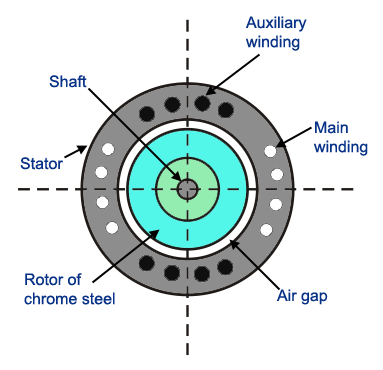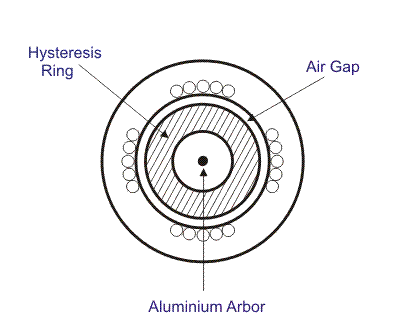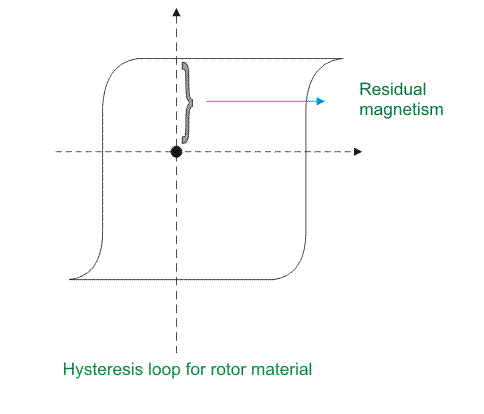## What is the Working Principle of Hysteresis Motor?

Starting behavior of a hysteresis motor is like a single phase induction motor and running behavior is same as a synchronous motor. Step by step its behavior can be realized in the working principle that is given below.
At the Starting Condition

When stator is energized with single phase AC supply, rotating magnetic field is produced in stator.
To maintain the rotating magnetic field the main and auxiliary windings must be supplied continuously at start as well as in running conditions.
At the starting, by induction phenomenon, secondary voltage is induced in the rotor by stator rotating magnetic field. Hence eddy current is generated to flow in the rotor and it develops rotor.
Thus eddy current torque is developed along with the hysteresis torque in the rotor. Hysteresis torque in the rotor develops as the rotor magnetic material is with high hysteresis loss property and high retentivity.
The rotor goes under the slip frequency before going to the steady state running condition.
So it can be said that when the rotor starts to rotate with the help of these eddy current torque due to induction phenomenon, it behalves like a single phase induction motor.

When the speed of the rotor reaches near about the synchronous speed, the stator pulls the rotor into synchronism.
At the condition of synchronism, the relative motion between stator field and rotor field vanishes. So there is no further induction phenomenon to continue. Hence no eddy current to generate in the rotor. Thus the torque due to eddy-currents vanishes.
At the time of rotor’s rotation at the synchronous speed, rotating magnetic field flux in the stator produces poles on the rotor by induction; they are named as north (N) and south (S) poles. Thus rotor behaves as a permanent magnet having rotor axis as the induced magnetic axis.
For high residual magnetism or retentivity the rotor pole strength remains sustainable or unchanged. Again higher the retentivity, higher is the hysteresis torque and the hysteresis torque is independent of the rotor speed always. The high retentivity enables the continuous magnetic locking between stator and rotor and thus the motor rotates at synchronous speed.
The maximum work done to establish the hysteresis losses under the magnetization cycle in the rotor is equal to the surface area inside B – H hysteresis curve.
In lower load torque, the needed work done to rotate the rotor is equal to maximum magnetizing work of hysteresis phenomenon available already in the rotor. So induced magnetic pole axis always follows the rotating magnetic field axis of stator without any lag angle.
But when the load torque is sufficiently high, the maximum magnetizing work in rotor by hysteresis phenomenon cannot fulfill the work done needed to rotate the rotor.
So the induced magnetic field axis or rotor pole axis lags the rotating magnetic field axis of the stator at an angle δh. Hence the rotor pole axis tries to catch up the stator magnetic field axis.
If the load torque is increased, this lagging angle will be increased up to δmax before dropping below the synchronous condition.
The rotor poles are attracted towards the moving stator poles and runs at synchronous speed.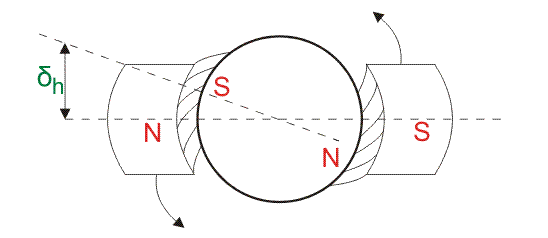As there is no slip at steady state running condition, only hysteresis torque is present to keep the rotor running at synchronous speed and it behaves like a synchronous motor.

What is Hysteresis Power Loss,
Ph in Hysteresis Motor?
Hysteresis power loss in the rotor of the hysteresis motor is given by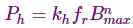Where,
fr is the frequency of flux reversal in the rotor (Hz)
Bmax is the maximum value of flux density in the air gap (T)
Ph is the heat-power loss due to hysteresis (W)
kh is the hysteresis constant

### What is the Equation of Hysteresis Torque in the Hysteresis Motor?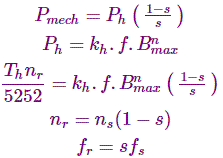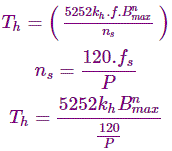From the equation of the hysteresis torque, it is clear that hysteresis torque is independent of frequency and speed.

## What is the Torque-Speed Characteristic of Hysteresis Motor?

Torque – speed characteristics of hysteresis motor is given below.
We know that constant Hysteresis Torque occurs in the hysteresis motor. This constant valued torque allows the motor to synchronize any load it can accelerate.
The normal operating range is mentioned with dark vertical line.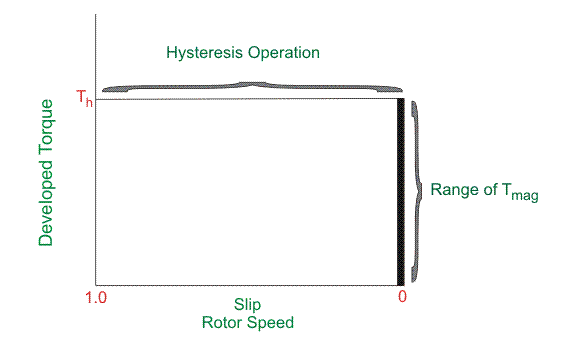## What is the Speed-Torque Characteristics?

The speed – torque characteristics of a hysteresis motor is shown below.
The torque is almost constant from starting to running condition. At starting condition the starting torque is the eddy current torque along with the hysteresis torque. But in the running condition net running torque means only the hysteresis torque.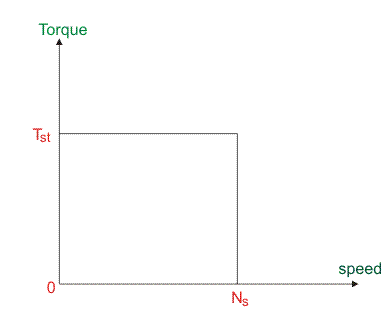## What is the Starting Torque of the Hysteresis Motor?

Suppose ΦS is the stator flux at synchronous speed.Φr is the rotor flux. Due to hysteresis effect, Φr lags ΦS at an angle α.
Then the starting torque produced is given by
TS = K.
ΦS× Φr × sinα.
K is the proportional constant.

## What are the Types of Hysteresis Motors?

There are various types of hysteresis motor by construction. They are
1.  Cylindrical hysteresis motors: It has cylindrical rotor.
2.  Disk hysteresis motors: It has annular ring shaped rotor.
3.  Circumferential – field hysteresis motor: It has rotor supported by a ring of non magnetic material with zero magnetic permeability.
4.  Axial – field hysteresis motor: It has rotor supported by a ring of magnetic material with infinite magnetic permeability.

What are the Advantages of Use of Hysteresis Motor?
The main advantages of hysteresis motor are given below.
As no teeth and no winding in rotor, no mechanical vibrations take place during its operation.
Its operation is quiet and noiseless as there is no vibration.
It is suitable to accelerate inertia loads.
Multispeed operation can be achieved by employing gear train.

What are the Disadvantages of use of Hysteresis Motor?
The disadvantages of hysteresis motor are given below.
Hysteresis motor has poor output that is one – quarter of output of an induction motor with same dimension.
Low efficiency
Low torque.
Low power factor
This type of motor is available in very small size only.

What are the Applications of Hysteresis Motors?
They are widely used in
1.  Sound producing equipments,
2.  Sound recording instruments,
3.  High quality record players,
4.  Timing devices
5.  Electric clocks,
6.  Teleprinters.